## How to Solve and Calculate for a Dimensionless Pressure Drop, Oil Rate, Oil FVF, Oil Viscosity in Well Testing | The Calculator Encyclopedia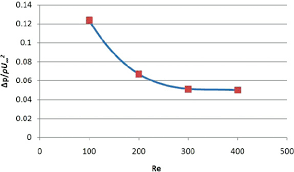The image above represents dimensionless pressure drop.

To compute for the dimensionless pressure drop, five essential parameters are needed and these parameters are Permeability-Thickness Product (kh), Pressure Drop (ΔP), Oil Rate (Qo), Oil Viscosity (μo) and Oil FVF (Bo).

The formula for calculating dimensionless pressure drop:

PD = khΔP / 141.2QoBoμo

Where;

PD = Dimensionless Pressure Drop
kH = Permeability-Thickness Product
ΔP = Pressure Drop
Qo = Oil Rate
μo = Oil Viscosity
Bo = Oil FVF

Let’s solve an example;
Find the dimensionless pressure drop with a permeability-thickness product of 70, a pressure drop of 30, an oil rate of 49, an oil viscosity of 19 and oil FVF of 31.

This implies that;

kh = Permeability-Thickness Product = 20
ΔP = Pressure Drop = 30
Qo = Oil Rate = 49
μo = Oil Viscosity = 19
Bo = Oil FVF = 31

PD = khΔP / 141.2QoBoμo
PD = 20 x 30/141.2 x 49 x 31 x 19
PD = 20 x 30/4075173.19
PD = 600/4075173.19
PD = 0.000147

Therefore, the dimensionless pressure drop is 0.000147 psi.

Calculating the Permeability-Thickness Product when Dimensionless Pressure Drop, Pressure Drop, Oil Rate, Oil Viscosity and Oil FVF.

kh = PD x 141.2QoBoμo / ΔP

Where;

kh = Permeability-Thickness Product
PD = Dimensionless Pressure Drop
ΔP = Pressure Drop
Qo = Oil Rate
μo = Oil Viscosity
Bo = Oil FVF

Let’s solve an example;
Find the permeability-thickness product with a dimensionless pressure drop of 28, a pressure drop of 16, an oil rate of 21, an oil viscosity of 18 and oil FVF of 24 .

This implies that;

PD = Dimensionless Pressure Drop = 28
ΔP = Pressure Drop =16
Qo = Oil Rate = 21
μo = Oil Viscosity = 18
Bo = Oil FVF = 24

kh = PD x 141.2QoBoμo / ΔP
kh = 28 x 141.2 x 21 x 24 x 18 / 16
kh = 35867059.8 / 16
kh = 2241691.2

Therefore, the permeability-thickness product is 2241691.2.

Calculating the Pressure Drop when Dimensionless Pressure Drop, Permeability-Thickness Product, Oil Rate, Oil Viscosity and Oil FVF.

ΔP = PD x 141.2QoBoμo / kh

Where;

ΔP = Pressure Drop
PD = Dimensionless Pressure Drop
kh = Permeability-Thickness Product
Qo = Oil Rate
μo = Oil Viscosity
Bo = Oil FVF

Let’s solve an example;
Find the pressure drop with a dimensionless pressure drop of 38, a permeability-thickness product of 24, an oil rate of 11, an oil viscosity of 8 and oil FVF of 12.

This implies that;

PD = Dimensionless Pressure Drop = 38
kh = Permeability-Thickness Product = 24
Qo = Oil Rate = 11
μo = Oil Viscosity = 8
Bo = Oil FVF = 12

ΔP = PD x 141.2QoBoμo / kh
ΔP = 38 x 141.2 x 11 x 12 x 8 / 24
ΔP = 5666073.6 / 24
ΔP = 236086.4

Therefore, the pressure drop is 236086.4.

## How to Calculate and Solve for Semi-Log Pressure-Time Slope, Permeability-Thickness Product, Oil Rate, Oil FVF, Oil Viscosity in Well Testing | Nickzom Calculator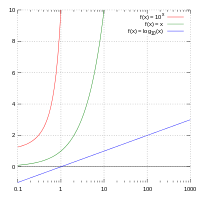The image above represents semi-log pressure-time slope.

To compute for the semi-log pressure-time slope, four essential parameters are needed and these parameters are Permeability-Thickness Product (kh), Oil Rate (Qo), Oil Viscosity (μo) and Oil FVF (Bo).

The formula for calculating the semi-log pressure-time slope:

m = 162.6QoBoμo / kh

Where;

m = Semi-Log Pressure-Time Slope
kh = Permeability-Thickness Product
Qo = Oil Rate
μo = Oil Viscosity
Bo = Oil FVF

Let’s solve an example;
Find the semi-log pressure-time slope, when permeability-thickness product is 12, oil rate is 17, oil viscosity is 21 and oil FVF is 13.7.

This implies that;

kh = Permeability-Thickness Product = 12
Qo = Oil Rate = 17
μo = Oil Viscosity = 21
Bo = Oil FVF = 13.7

m = 162.6QoBoμo / kh
m = 162.6 x 17 x 13.7 x 21 / 12
m = 795260.34 / 12
m = 66271.69

Therefore, the semi-log pressure-time slope is 66271.69.

Calculating the Permeability-Thickness Product when Semi-Log Pressure-Time Slope, Oil Rate, Oil Viscosity and Oil FVF is Given.

kh = 162.6QoBoμo / m

Where;

kh = Permeability-Thickness Product
m = Semi-Log Pressure-Time Slope
Qo = Oil Rate
μo = Oil Viscosity
Bo = Oil FVF

Let’s solve an example;
Find the permeability-thickness product, when semi-log pressure-time slope is 90, oil rate is 23, oil viscosity is 27 and oil FVF is 14.

This implies that;

m = Semi-Log Pressure-Time Slope = 90
Qo = Oil Rate = 23
μo = Oil Viscosity = 27
Bo = Oil FVF = 14

kh = 162.6QoBoμo / m
kh = 162.6 x 23 x 14 x 27 / 90
kh = 1413644.4 / 90
kh = 15707.16

Therefore, the permeability-thickness product is 15707.16.

Calculating the Oil Rate when Semi-Log Pressure-Time Slope, Permeability-Thickness Product, Oil Viscosity and Oil FVF is Given.

Qo = m x kh / 162.6Boo

Where;

Qo = Oil Rate
m = Semi-Log Pressure-Time Slope
kh = Permeability-Thickness Product
μo = Oil Viscosity
Bo = Oil FVF

Let’s solve an example;
Find the oil rate when semi-log pressure-time slope is 110, permeability-thickness product is 42, oil viscosity is 31 and oil FVF is 11.

This implies that;

m = Semi-Log Pressure-Time Slope = 110
kh = Permeability-Thickness Product = 42
μo = Oil Viscosity = 31
Bo = Oil FVF = 11

Qo = m x kh / 162.6Boμo
Qo = 110 x 42 / 162.6 x 11 x 31
Qo = 4620 / 55446.6
Qo = 0.0833

Therefore, the oil rate is 0.0833.

## How to Calculate and Solve for Well, External and Dimensionless Radius | Nickzom Calculator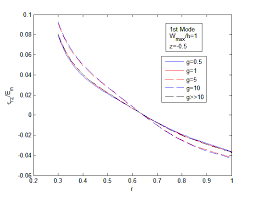The image above represents dimensionless radius.

To compute for the dimensionless radius, two essential parameters are needed and these parameters are external radius (re) and well radius (rw).

The formula for calculating the dimensionless radius:

rD = re / rw

Where;

Let’s solve an example;
Given that the external radius is 18 and the well radius is 16.

This implies that;

re = External Radius = 18
rw = Well Radius = 16

rD = re / rw
rD = 18 / 16
rD = 1.125

Therefore, the dimensionless radius is 1.125.

re = rD x rw

Where;

Let’s solve an example;
Given that the dimensionless radius is 9 and the well radius is 20.

This implies that;

rD = Dimensionless Radius = 9
rw = Well Radius = 20

re = rD x rw
re = 9 x 20
re = 180

Therefore, the external radius is 180.

## How to Calculate and Solve for Well Bore Storage Factor, Fluid Density and Well Annulus Cross-Sectional Area in Well Testing | The Calculator Encyclopedia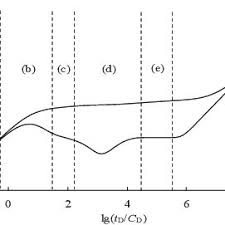The image above represents the well bore storage factor.

To compute for the well bore storage factor, two essential parameters are needed and these parameters are Well Annulus Cross-Sectional Area (Ao) and Fluid Density (ρ).

The formula for calculating the well bore storage factor:

C = 144Ao / 5.615ρ

Where;

C = Well Bore Storage Factor
Ao = Well Annulus Cross-Sectional Area
ρ = Fluid Density

Let’s solve an example;
Find the well bore storage factor when the well annulus cross-sectional area is 54 and fluid density is 42.

This implies that;

Ao = Well Annulus Cross-Sectional Area = 54
ρ = Fluid Density = 42

C = 144Ao / 5.615ρ
C = 144 x 54 / 5.615 x 42
C = 7776/235.83
C = 32.97

Therefore, the well bore storage factor is 32.97.

Calculating the Well Annulus Cross-Sectional Area when the Well bore Storage Factor and the Fluid Density is Given.

Ao = C x 5.615ρ / 144

Where;

Ao = Well Annulus Cross-Sectional Area
C = Well Bore Storage Factor
ρ = Fluid Density

Let’s solve an example;
Find the well annulus cross-sectional area when the well bore storage factor is 34 and fluid density is 24.

This implies that;

C = Well Bore Storage Factor = 34
ρ = Fluid Density = 24

Ao = C x 5.615ρ / 144
Ao = 34 x 5.615 x 24 / 144
Ao = 4581.84 / 144
Ao = 31.81

Therefore, the well annulus cross-sectional area is 31.81.

## How to Calculate and Solve for Total Production Time, Stabilized Well Flow, Cumulative Production before Shut-In | Well Testing | Nickzom Calculator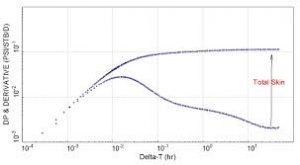The image represents the total production time.

To compute for the total production time, two essential parameters are needed and these parameters are Cumulative Production before Shut-In (Np) and Stabilized Well Flow (Qo).

The formula for calculating the total production time:

tp = 24Np / Qo

Where:

tp = Total Production Time
Np = Cumulative Production before Shut-In
Qo = Stabilized Well Flow

Let’s solve an example;
Find the total production time when the cumulative production before Shut-In is 24 and the stabilized well flow is 43.

This implies that;

Np = Cumulative Production before Shut-In = 24
Qo = Stabilized Well Flow = 43

tp = 24Np / Qo
tp = 24 x 24 / 43
tp = 576 / 43
tp = 13.39

Therefore, the total production time is 13.39 hrs.

## How to Calculate and Solve for the Radius of Investigation in Well Testing | The Calculator Encyclopedia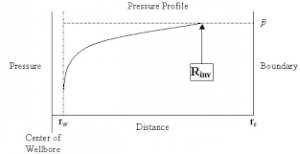The image above represents radius of investigation.

To compute for the radius of investigation, five essential parameters are needed and these parameters are permeability (k), porosity (φ), viscosity (μ), time (t) and total compressibility (CT).

The formula for calculating the radius of investigation:

rinv = 0.0325 √[Kt / φμCT]

Where:

K = Permeability
φ = Porosity
μ = Viscosity
t = Time
CT = Total Compressibility

Let’s solve an example;
Find the radius of investigation when the permeability is 25, porosity is 18, viscosity is 12, time is 22 and total compressibility is 37.

This implies that;

K = Permeability = 25
φ = Porosity =18
μ = Viscosity = 12
t = Time = 22
CT = Total Compressibility = 37

rinv = 0.0325 √[Kt / φμCT]
rinv = 0.0325 √[25 x 22 / 18 x 12 x 37]
rinv = 0.0325 √[25 x 22 / 7992]
rinv = 0.0325 √[550 / 7992]
rinv = 0.0325 √[0.068]
rinv = 0.0325 [0.26]
rinv = 0.0085

Therefore, the radius of investigation is 0.0085 ft.

## How to Calculate and Solve for Infinite Acting Period | The Calculator Encyclopedia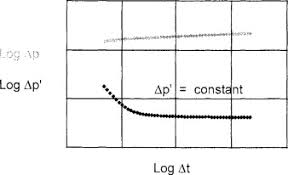The image represents the infinite acting period.

To compute the infinite acting period, six essential parameters are needed and these parameters are permeability (k), porosity (φ), well drainage area (A), viscosity (μ), Dimensionless Time to End of Infinite Acting Period ((tDA)eia) and total compressibility (CT).

The formula for calculating the infinite acting period:

teia = [φ μ CT A / 0.000263K] (tDA)eia

Where;

teia = Infinite Acting Period
K = Permeability
φ = Porosity
A = Well Drainage Area
μ = Viscosity
(tDA)eia = Dimensionless Time to End of Infinite Acting Period
CT = Total Compressibility

Let’s solve an example;
Given that the permeability is 21, porosity is 15, well drainage area is 32, viscosity is 26, dimensionless time to end of infinite acting period is 44 and total compressibility is 34.
Find the infinite acting period?

This implies that;

K = Permeability = 21
φ = Porosity = 15
A = Well Drainage Area = 32
μ = Viscosity = 26
(tDA)eia = Dimensionless Time to End of Infinite Acting Period = 44
CT = Total Compressibility = 34

teia = [φ μ CT A / 0.000263K] (tDA)eia
teia = [15 x 26 x 34 x 32 / 0.000263 x 21] 44
teia = [424320 / 0.005523] 44
teia = [76827810.972] 44
teia = 3380423682.78

Therefore, the infinite acting period is 3380423682.78 s.

## How to Calculate and Solve for Dimensionless Time in Well Testing | The Calculator Encyclopedia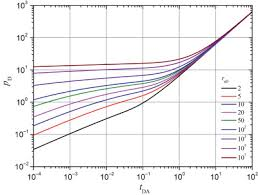The image above represents dimensionless time.

To compute for the dimensionless time, six essential parameters are needed and these parameters are permeability (k), porosity (φ), viscosity (μ), well radius (rw), minimum transient time (t) and total compressibility (CT).

The formula for calculating dimensionless time:

tD = 0.000263kt / φμCTr

Where;

tD = Dimensionless Time
k = Permeability
φ = Porosity
μ = Viscosity
t = Minimum Transient Time
CT = Total Compressibility

Let’s solve an example
Find the dimensionless time when the permeability is 13, porosity is 11.5, viscosity is 10.5, well radius is 24, minimum transient time is 17 with a total compressibility of 28.

This implies that;

k = Permeability = 13
φ = Porosity = 11.5
μ = Viscosity = 10.5
rw = Well Radius = 24
t = Minimum Transient Time = 17
CT = Total Compressibility = 28

tD = 0.000263kt / φμCTr
tD = 0.000263 x 13 x 1711.5 x 10.5 x 28 x 24²
tD = 0.000263 x 13 x 1711.5 x 10.5 x 28 x 576
tD = 0.000263 x 13 x 171947456
tD = 0.0581231947456
tD = 2.9845e-8

Therefore, the dimensionless time is 2.98

Nickzom Calculator – The Calculator Encyclopedia is capable of calculating the dimensionless time.

To get the answer and workings of the dimensionless time using the Nickzom Calculator – The Calculator Encyclopedia. First, you need to obtain the app.

You can get this app via any of these means:

To get access to the professional version via web, you need to register and subscribe for NGN 1,500 per annum to have utter access to all functionalities.
You can also try the demo version via https://www.nickzom.org/calculator

Apple (Paid) – https://itunes.apple.com/us/app/nickzom-calculator/id1331162702?mt=8
Once, you have obtained the calculator encyclopedia app, proceed to the Calculator Map, then click on Petroleum under EngineeringNow, Click on Well Testing under Petroleum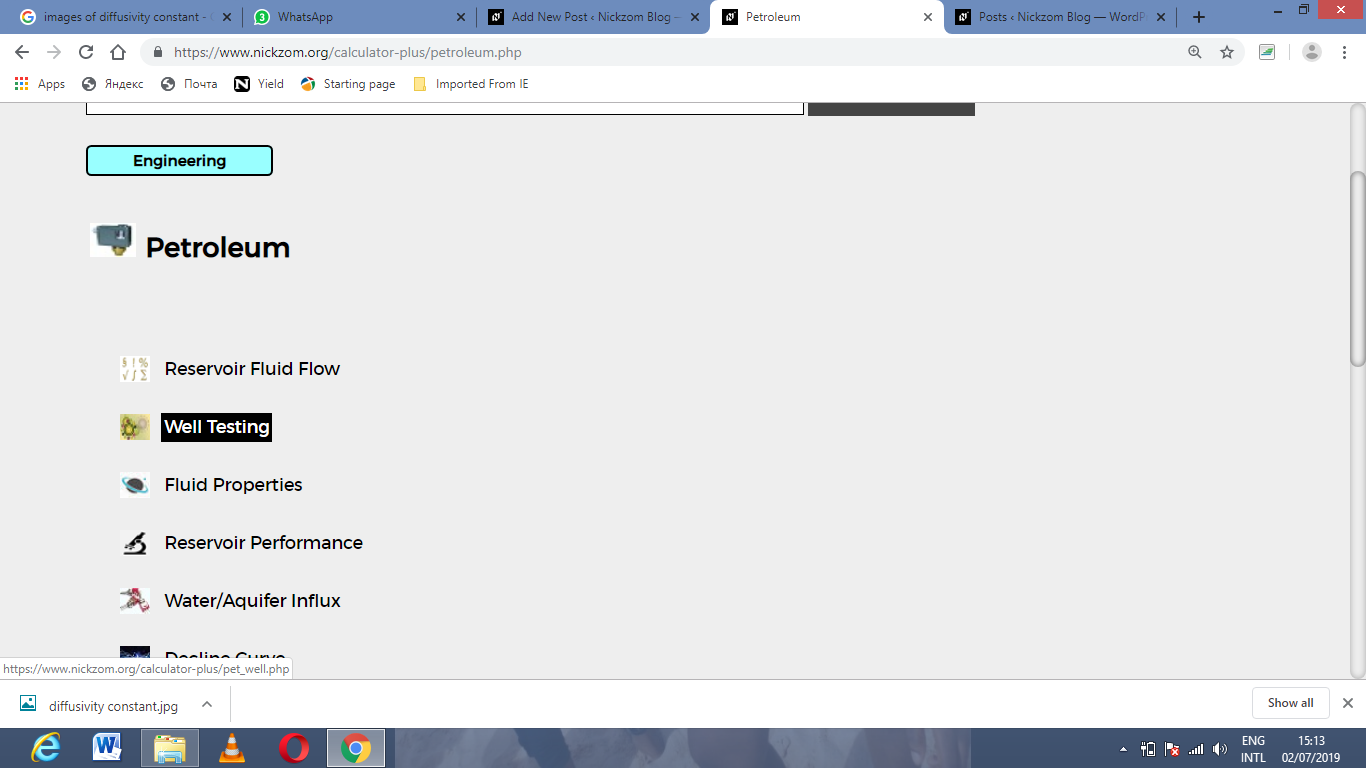Now, Click on Dimensionless Time under Well Testing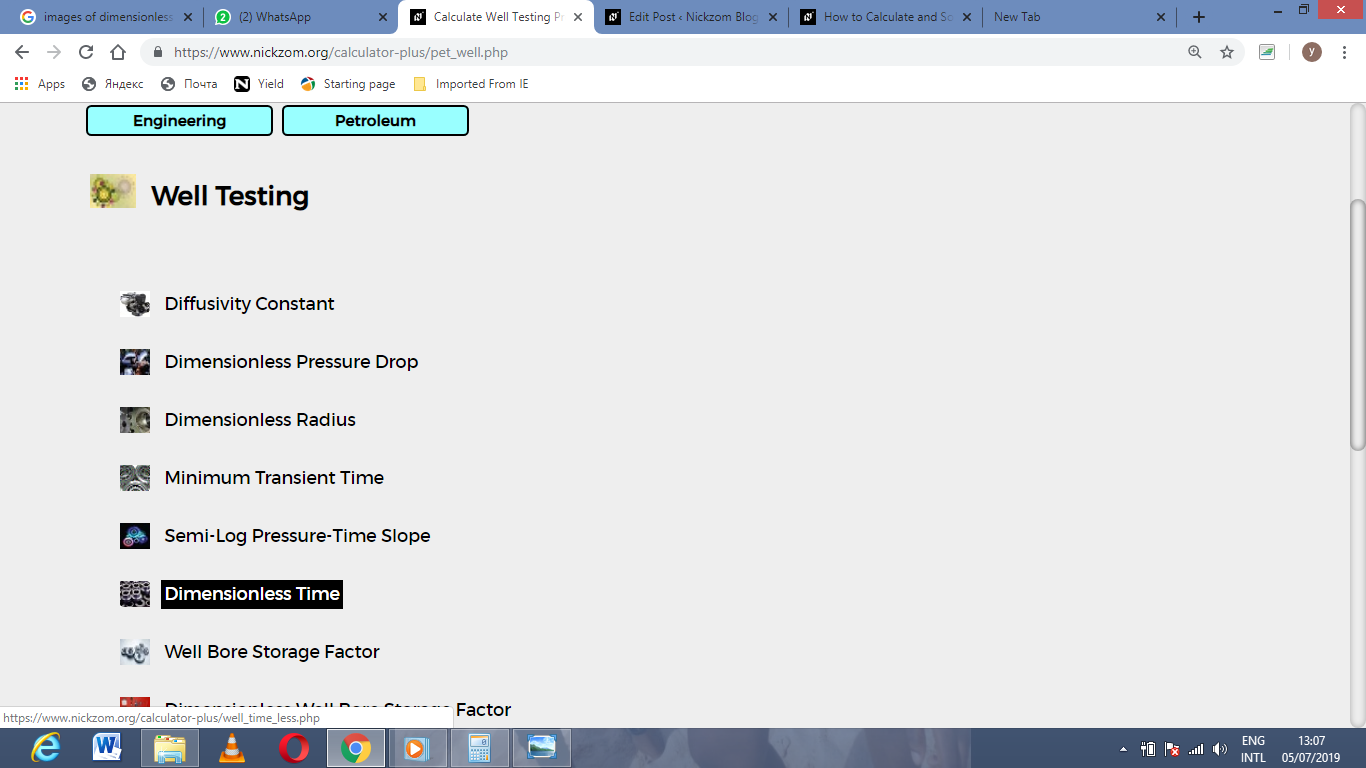The screenshot below displays the page or activity to enter your value, to get the answer for the dimensionless time according to the respective parameter which are the permeability (k), porosity (φ), viscosity (μ), well radius (rw), minimum transient time (t) and total compressibility (CT).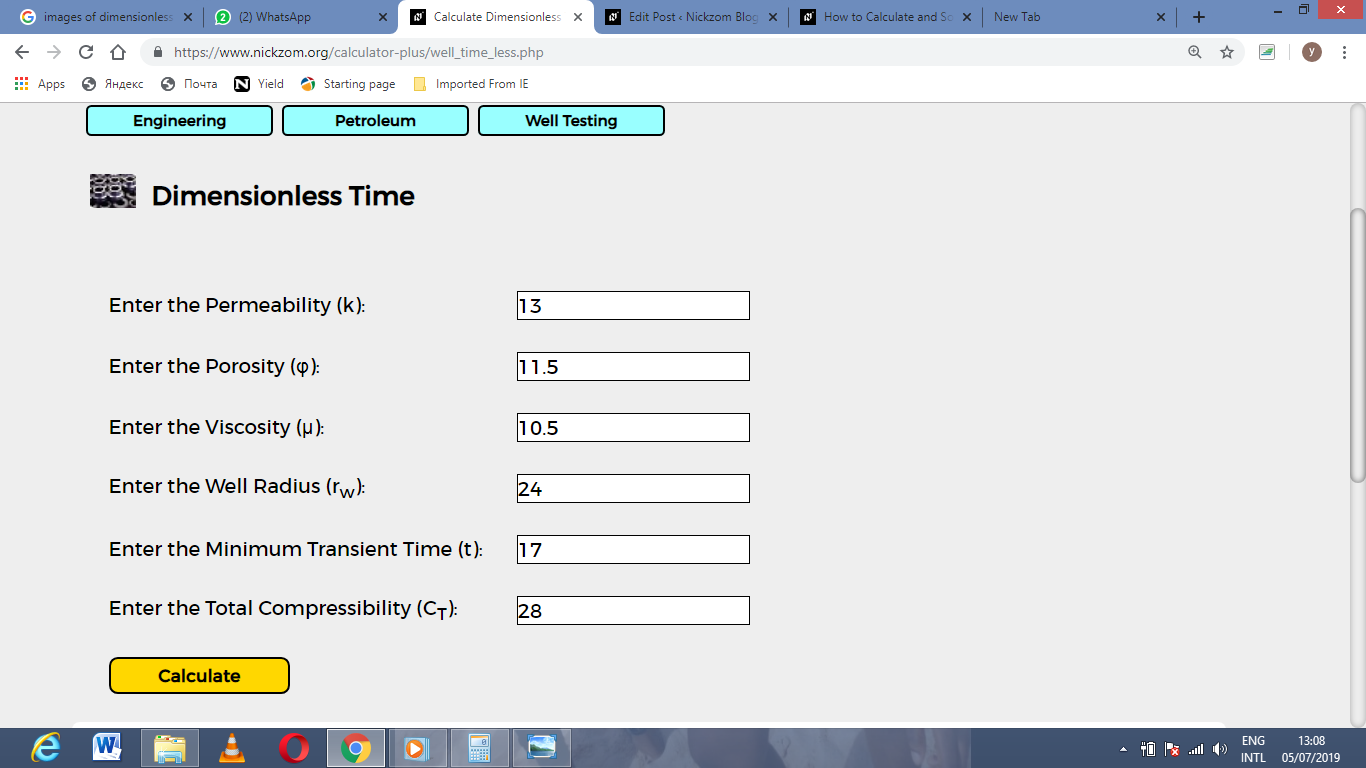Now, enter the value appropriately and accordingly for the parameter as required by the permeability (k) is 13, porosity (φ) is 11.5, viscosity (μ) is 10.5, well radius (rw) is 24, minimum transient time (t) is 17 and total compressibility (CT) is 28.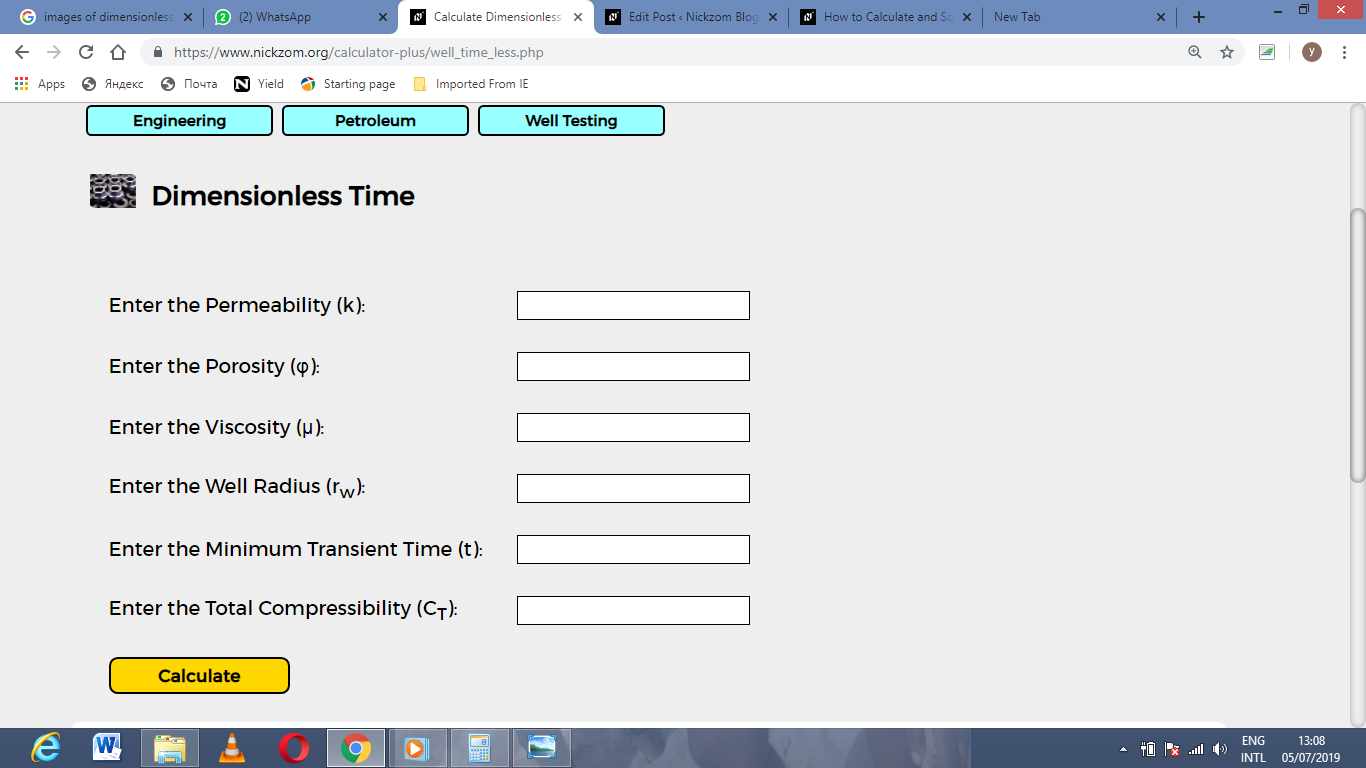Finally, Click on Calculate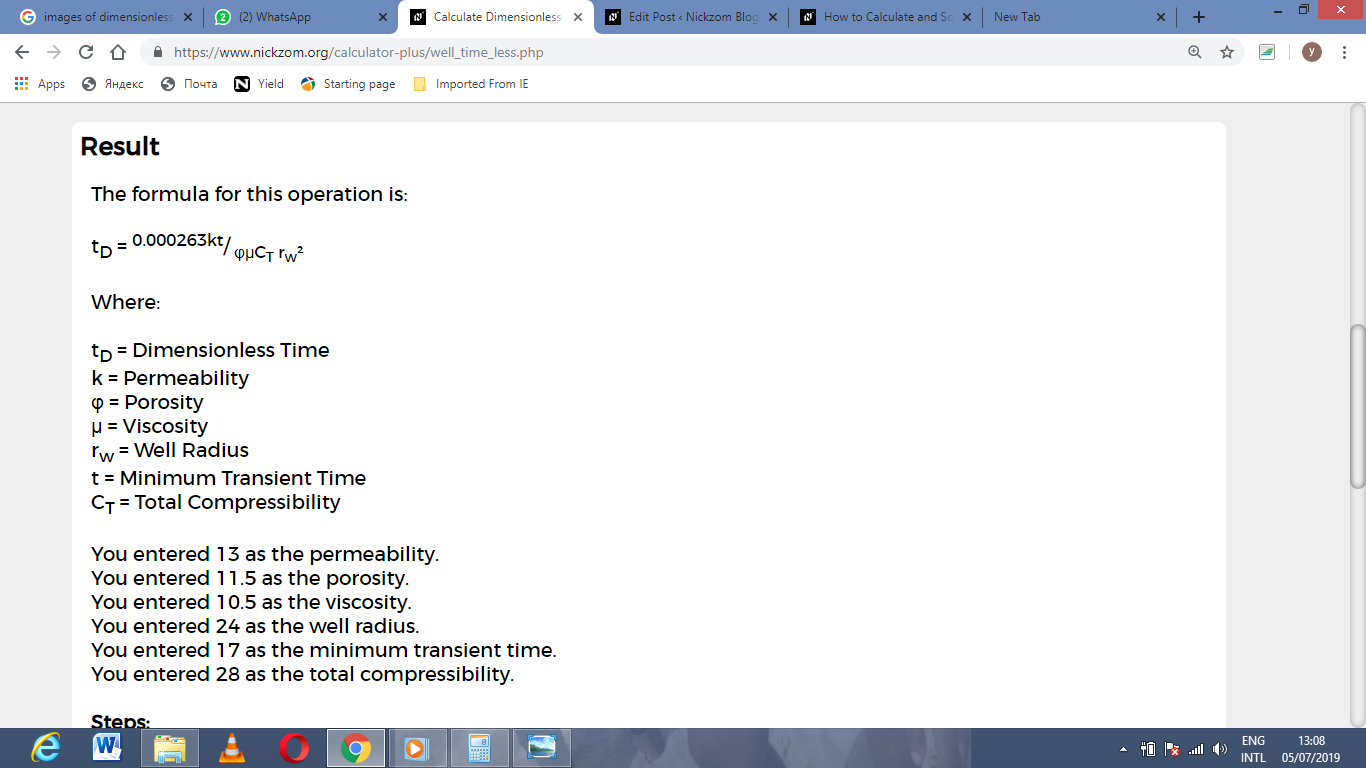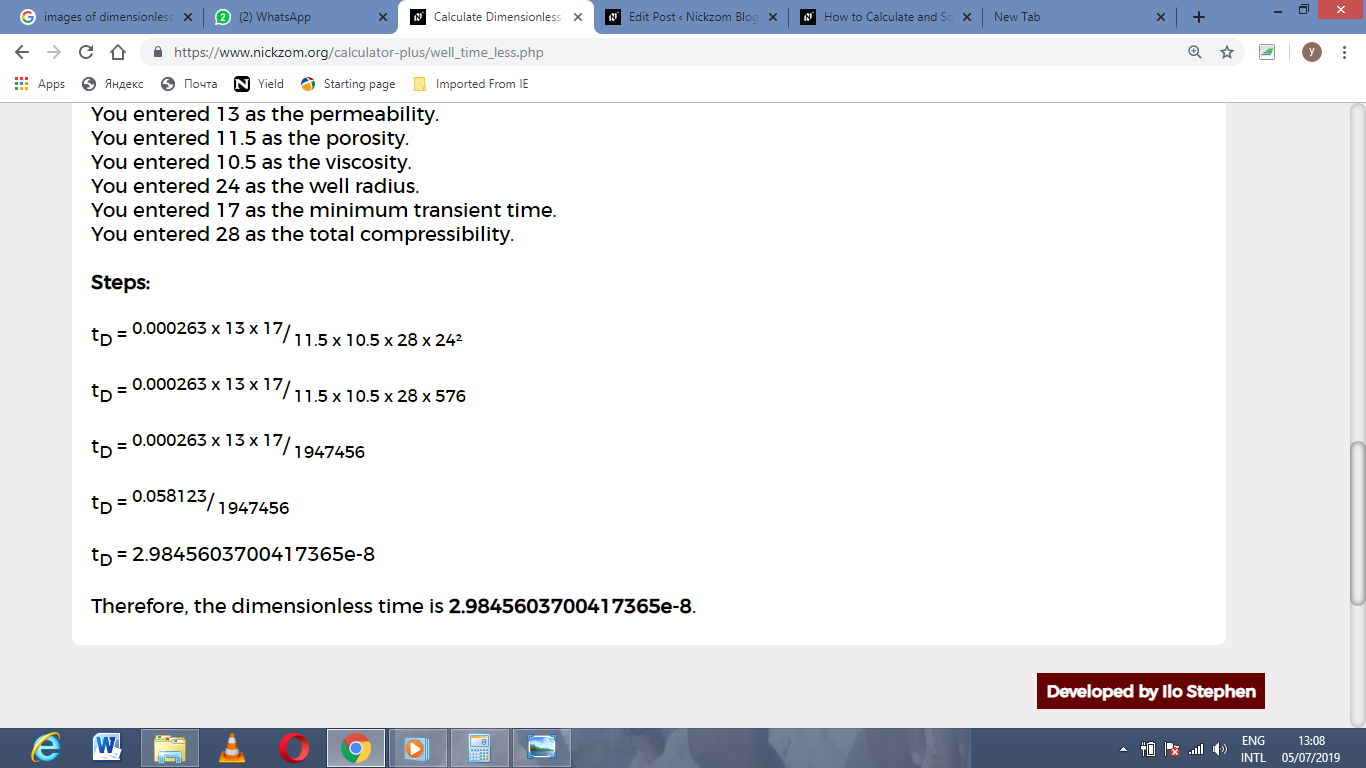As you can see from the screenshot above, Nickzom Calculator– The Calculator Encyclopedia solves for the dimensionless time and presents the formula, workings and steps too.

## How to Calculate and Solve for Minimum Transient Time in Well Testing | The Calculator Encyclopedia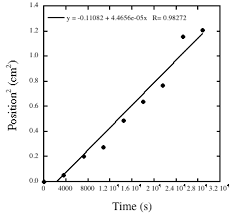The image above represents minimum transient time.

To compute for the minimum transient time, five essential parameters are needed and these parameters are permeability (k), porosity (φ), reservoir radius (r), viscosity (μ) and total compressibility (CT).

The formula for calculating the minimum transient time:

t = 9.48 x 104 φ CT r² / k

Where;

t = Minimum Transient Time
k = Permeability
φ = Porosity
μ = Viscosity
CT = Total Compressibility

Let’s solve an example;
Given that the permeability is 11, porosity is 14, reservoir radius is 7, viscosity is 13 and total compressibility is 22.
Find the minimum transient time?

This implies that;

k = Permeability = 11
φ = Porosity = 14
r = Reservoir Radius = 7
μ = Viscosity = 13
CT = Total Compressibility = 22

t = 9.48 x 104 φ CT r² / k
t = 9.48 x 104 x 14 x 22 x 7² / 11
t = 9.48 x 104 x 14 x 22 x 49 / 11
t = 1430721600 / 11
t = 130065600

Therefore, the minimum transient time is 13006500.

## How to Calculate and Solve for Diffusivity Constant | Well Testing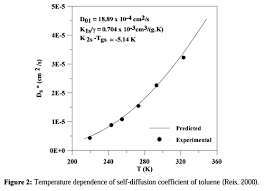The image above represents diffusivity constant.

To compute for the diffusivity constant, four essential parameters are needed and these parameters are pemerability (k), porosity (φ), viscosity (μ) and total compressibility (CT).

The formula for calculating diffusivity constant:

η = 0.000263k / φμCT

Where;

η = Diffusivity Constant
k = Permeability
φ = Porosity
μ = Viscosity
CT = Total Compressibility

Let’s solve an example;
Find the diffusivity constant where the permeability is 12, porosity is 9, viscosity is 7 and total compressibility is 11.

This implies that;

k = Permeability = 12
φ = Porosity = 9
μ = Viscosity = 7
CT = Total Compressibility = 11

η = 0.000263k /  φμCT
η = 0.000263 x 12 / 9 x 7 x 11
η = 0.003156 / 693
η = 0.000004554112554112554

Therefore, the diffusivity constant is 0.000004554112554112554.

Calculating the Permeability when the Diffusivity Constant, Porosity, Viscosity and Total Compressibility is Given.

k = n x φμCT / 0.000263

Where;

k = Permeability
η = Diffusivity Constant
φ = Porosity
μ = Viscosity
CT = Total Compressibility

Let’s solve an example;
Find the permeability where the diffusivity constant is 24, porosity is 10, viscosity is 5 and total compressibility is 13.

This implies that;

η = Diffusivity Constant = 24
φ = Porosity = 10
μ = Viscosity = 5
CT = Total Compressibility = 13

k = n x φμCT / 0.000263
k = 24 x 10 x 5 x 13 / 0.000263
k = 15600 / 0.000263
k = 59315589.3536

Therefore, the permeability is 59315589.3536.

Calculating the Porosity when the Diffusivity Constant, Permeability, Viscosity and Total Compressibility is Given.

φ = 0.000263k / nμCT

Where;

φ = Porosity
k = Permeability
η = Diffusivity Constant
μ = Viscosity
CT = Total Compressibility

Let’s solve an example;
Find the porosity where the diffusivity constant is 28, permeability is 8, viscosity is 12 and total compressibility is 18.

This implies that;

k = Permeability = 8
η = Diffusivity Constant = 28
μ = Viscosity = 12
CT = Total Compressibility = 18

φ = 0.000263k / nμCT
φ = 0.000263 x 8 / 28 x 12 x 18
φ = 0.002104 / 6048
φ = 3.478e-7

Therefore, the porosity is 3.47e-7.